# Lebesgue function

A function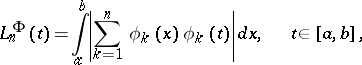where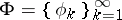is a given system of functions, orthonormal with respect to the Lebesgue measure on the interval,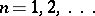. Lebesgue functions are defined similarly in the case when an orthonormal system is specified on an arbitrary measure space. One has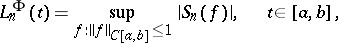where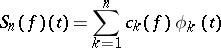is the-th partial sum of the Fourier series ofwith respect to. In the case whenis the trigonometric system, the Lebesgue functions are constant and reduce to the Lebesgue constants. They were introduced by H. Lebesgue.